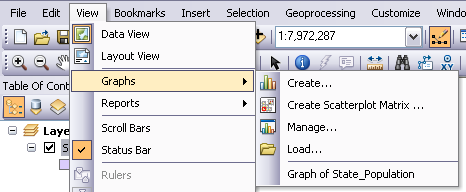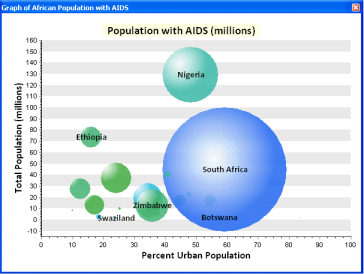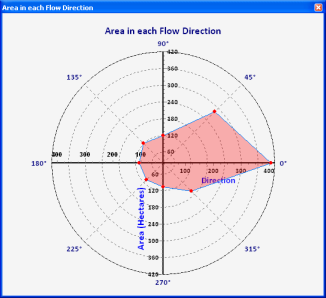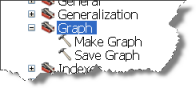What's new for graphing in ArcGIS 10
New graphing in ArcGIS 10

You can create graphs in ArcGIS Desktop applications to visualize and explore data. With a graph, it is easy to quickly compare features and gain insight into the functional relationship between the features, thereby visualizing the distribution, trends, and patterns in the data that otherwise would be difficult to see.## New graph types

In ArcGIS 10, three new graph types are supported for creating graphs.

### Bubble graphs

Bubble graphs are like scatterplot graphs, except that they allow an additional size variable to be incorporated into the graph. Essentially, this is like charting three variables in two dimensions. The bubbles are represented by markers that vary in size, indicating relative importance. In the example below, three variables—Total Population (y-axis), Percentage of Urban population (x-axis), and Population with AIDS (size of bubble)—are graphed.### Polar graph

A polar graph is essentially a line graph drawn on a circular form that can show trends in data values by means of angles. Polar graphs are useful primarily in mathematical and statistical applications. These graphs are used to display variables that vary based on direction. You can plot multiple datasets on the polar graph, where each dataset is represented by a single line. In the example below, the total area in each flow direction of water has been represented on a polar graph. In this case, most of the area has a slope from west to east, which means that in case of a rainfall event water will tend to flow east.### Bar min and max graph

Bar min and max graphs are used to display the minimum and maximum values associated with your geographic data. For example, you can display the minimum and maximum streamflow discharge values at different gauging stations at a particular point in time along a stream or river. Like a bar graph, the bar min and max graph is used to display discrete data in separate columns, except that the columns are not grounded on the x-axis but rather start at the minimum value and end at the maximum value. Bar min and max graphs compare amounts to each other but add the ability to visualize the minimum and maximum value of the series.

## Geoprocessing tools for creating and saving graphs

In ArcGIS 10, you can create graphs using geoprocessing tools found in the Graph toolset in the Data Management toolbox. These tools (Make Graph and Save Graph) can be used one at a time or chained together in a model or script to create a graph of the desired type.